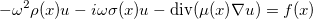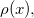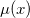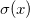# How physical indexes are implemented in Montjoie

In equations solved by Montjoie, for instance Helmholtz equation :The coefficientsandare the so-called physical indexes and depend on the position x. The physical indexes can be constant on the different domains of the mesh, but can also vary continuously inside each domain. An index can be scalar, vectorial, tensorial, each scalar component is either a constant (on the considered domain) or a varying field (object of type PhysicalVaryingMedia). In the case of a varying field, the constant stored in the object PhysicalVaryingMedia is used to specify a value of the physical index at infinity (used for PML or absorbing boundary condition).

### Public attributes of class PhysicalVaryingMedia

 index_regular varying field defined through values on a regular grid index_sinus varying field defined as a product of sine functions index_mesh varying field defined through values on nodal points of the mesh index_radial varying field defined through values at different radii r

### Public methods of class RectangleCutOff

 Init initializes the boundaries of the cut-off function InsideBox returns true if the point is inside the definition domain of the cut-off function ApplyCutOff applies cut-off function to a value

### Public methods of class PhysicalSinusoidalMedia

 IsComplex returns true if the physical index contains values with non-null imaginary part GetInverse inverts the field, so that 1/rho is returned instead of rho Init_Sine initializes the parameters of the sinusoidal field Mlt modifies the field such that alpha rho is returned instead of rho ComputeIndexAtPoint computes the field at a given point ComputeIndex computes the field on quadrature points of a mesh

### Public methods of class PhysicalRegularMedia

 Init_Regular initializes parameters of the field SetNoPeriodic the field is assumed to be non-periodic SetPeriodic the field is assumed to be periodic SetQuasiPeriodic the field is assumed to be quasi-periodic IsComplex returns true if the physical index contains values with non-null imaginary part GetInverse inverts the field, so that 1/rho is returned instead of rho Mlt modifies the field such that alpha rho is returned instead of rho Clear releases the memory used to store the field ReadFile reads the values of the field on the regular grid ComputeIndexAtPoint computes the field at a given point ComputeIndex computes the field on quadrature points of a mesh

### Public methods of class PhysicalMeshMedia

 IsComplex returns true if the physical index contains values with non-null imaginary part GetInverse inverts the field, so that 1/rho is returned instead of rho Mlt modifies the field such that alpha rho is returned instead of rho Clear releases the memory used to store the field GetMeshName returns the name of the file where the mesh is stored GetOrder returns the order of approximation used for nodal points of the mesh GetNbElt returns the number of elements of the mesh GetNbNodes returns the number of nodes on an element of the mesh GetValue returns the value of the field on a nodal point of the mesh GetGradient returns the gradient of the field on a nodal point of the mesh Init_Mesh initializes the parameters of the field defined on nodal points of a mesh RemoveGlobalElements removes elements that are located on other processors ReadFile reads the values of the field ComputeGradient computes the gradient of the field

### Public methods of class RadialVaryingMedia

 Init_Radial initializes the parameters of the radial field IsComplex returns true if the physical index contains values with non-null imaginary part GetInverse inverts the field, so that 1/rho is returned instead of rho Mlt modifies the field such that alpha rho is returned instead of rho Clear releases the memory used to store the field ComputeIndexAtPoint computes the field at a given point ComputeIndex computes the field on quadrature points of a mesh

### Public methods of class ScalarPhysicalIndice

 IsZero returns true if the physical index is equal to zero IsComplex returns true if the physical index contains values with non-null imaginary part IsVarying returns true if the physical index is varying, false if it is constant SetMediaType sets the type of varying index GetMediaType returns the type of varying index GetNbVaryingMedia returns the number of varying field GetVaryingMedia retrieves addresses of the varying fields stored in the index SetIdentity sets the index to one GetConstant returns the constant associated with the index SetConstant modifies the constant associated with the index Zero sets the index to 0 GetInverse inverts the index, so that 1/rho is returned instead of rho Mlt modifies the index such that alpha rho is returned instead of rho Clear releases the memory used to store the index GetCoefficient returns the index on a quadrature point of the mesh GetCoefGradient returns the gradient of the index on a quadrature point of the mesh SetInputVaryingMedia initializes a varying field from parameters of the data file SetInputData initializes the index from parameters of the data file

### Public methods of class VectorialPhysicalIndice

 IsZero returns true if the physical index is equal to zero IsComplex returns true if the physical index contains values with non-null imaginary part IsVarying returns true if the physical index is varying, false if it is constant SetMediaType sets the type of varying index GetMediaType returns the type of varying index GetNbVaryingMedia returns the number of varying field GetVaryingMedia retrieves addresses of the varying fields stored in the index SetIdentity sets the index to one GetConstant returns the constant associated with the index SetConstant modifies the constant associated with the index Zero sets the index to 0 Mlt modifies the index such that alpha rho is returned instead of rho Clear releases the memory used to store the index GetCoefficient returns the index on a quadrature point of the mesh GetCoefGradient returns the gradient of the index on a quadrature point of the mesh SetInputVaryingMedia initializes a varying field from parameters of the data file SetInputData initializes the index from parameters of the data file

### Public methods of class TensorPhysicalIndice

 IsZero returns true if the physical index is equal to zero IsComplex returns true if the physical index contains values with non-null imaginary part IsVarying returns true if the physical index is varying, false if it is constant SetMediaType sets the type of varying index GetMediaType returns the type of varying index SetAnisotropy sets the type of anisotropy (isotrope, orthotrope or anisotrope) GetAnisotropy returns the type of anisotropy (isotrope, orthotrope or anisotrope) GetNbVaryingMedia returns the number of varying field GetVaryingMedia retrieves addresses of the varying fields stored in the index SetIdentity sets the index to the identity matrix GetConstant returns the constant associated with the index SetConstant modifies the constant associated with the index Zero sets the index to 0 Mlt modifies the index such that alpha rho is returned instead of rho GetInverse inverts the index, so that rho^{-1} is returned instead of rho Clear releases the memory used to store the index MltMatrix applies the tensor to a vector GetCoefficient returns the index on a quadrature point of the mesh GetCoefGradient returns the gradient of the index on a quadrature point of the mesh SetInputVaryingMedia initializes a varying field from parameters of the data file SetInputData initializes the index from parameters of the data file

### Public methods of class ElasticPhysicalIndice

 IsComplex returns true if the physical index contains values with non-null imaginary part IsVarying returns true if the physical index is varying, false if it is constant SetMediaType sets the type of varying index GetMediaType returns the type of varying index GetAnisotropyType returns the type of anisotropy (isotrope, orthotrope or anisotrope) GetNbVaryingMedia returns the number of varying fields GetVaryingMedia retrieves addresses of the varying fields stored in the index SetIdentity sets the index to the identity elastic tensor GetConstant returns the constant associated with the index SetConstant modifies the constant associated with the index Zero sets the index to 0 Mlt modifies the index such that alpha rho is returned instead of rho GetInverse inverts the index, so that rho^{-1} is returned instead of rho Clear releases the memory used to store the index MltMatrix applies the tensor to a vector GetCoefficient returns the index on a quadrature point of the mesh MltMatrix applies the tensor to a matrix MltVector applies the tensor to a vector viewed as a matrix SetInputVaryingMedia initializes a varying field from parameters of the data file SetInputData initializes the index from parameters of the data file